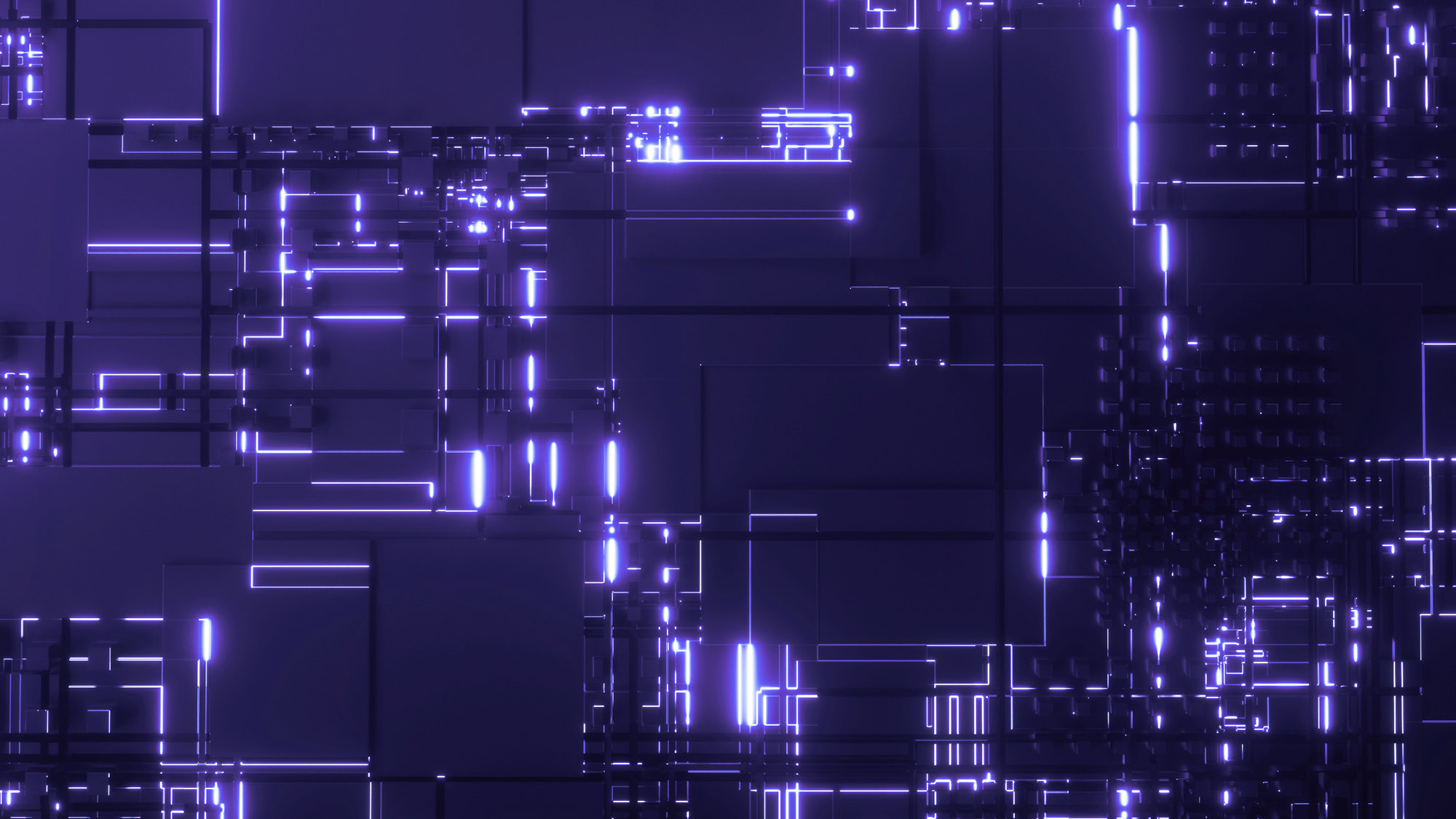Technical

# Quantum RAM: A New Quantum Milestone

Quantum RAM, also known as qRAM, is the quantum equivalent of classical RAM. The concept is fundamental for quantum information processing and data analysis, as well as for most quantum algorithms.###### Quantum RAM: A New Quantum Milestone

We see a future where we efficiently utilize quantum properties for machine learning and pattern recognition, anticipating quantum computers to revolutionize the field of artificial intelligence. However, most of these quantum algorithms are devised assuming that we already have a quantum equivalent of classical RAM, which, in reality, still has not been constructed yet. In this article, we will dive into what quantum RAM is, how it contrasts with its classical counterpart, and why we need it. Moreover, we will explore a proposed model of quantum RAM along with its limitations.

## What is Quantum RAM?

For computation not entirely based on the current input, we need an easily accessible memory device. This memory device should be readable (data can be fetched from the storage base) and writable (we can assign and reset values as per necessity). It acts as a relay between processor and data-store. For classical computers this job is carried out by Random Access Memory: aka. "RAM." RAM consists of three main components: input register, output register and memory cells. The input register is often termed an address register. A memory cell is the fundamental unit of computer memory and multiple memory cells form the memory. The input and output registers take entry and read out the corresponding data retrieved from the memory array, respectively.

As for quantum computers, we need a quantum mechanical version of RAM that would retrieve the data from the storage base not as bit-strings but as states. Thus, the input and output register in Quantum-RAM is qubits instead of bits. These qubits fetch a superposition of data ready to be used in quantum algorithms. The memory array can either be quantum or classical in nature depending on the application.

In general, there are two types of algorithms that require quantum RAM: the data is classical in nature and for the other, the data is inherently quantum in nature (for example: quantum chemistry simulation).

## Why do we need a qRAM?

The algorithmic speed-up that is achieved exclusively in quantum algorithms is the implication of entanglement and superposition of qubits. So, quantum devices compute by operating on states of qubits rather than bits of either 0s or 1s. When we want to work with data, whether classical or quantum in nature, they need to be encoded in the form of states of qubits. This allows them to be processed in a quantum machine,. For instance, for analyzing data in quantum machine learning, the data needs to be pre-processed and mapped to any quantum property. Then, by a RAM-call, the superposition of data is retrieved for operation. In technical terms, the data register (output) will return a superposition of memory cells in the address register (input). And thus instead of bits, the data register and address register in qRAMs need to be qubits. The following theoretical framework provides a conceptual architecture for constructing a quantum RAM:

Suppose, we have  N=2^n  memory cells arranged at the end of the following bifurcation graph, where n is the number of levels: